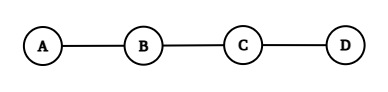Problem title
Difficulty
Avg time to solve

Lucky Group
Ninja
80 mins
Biconnected Components
Hard
70 mins
Morning Walk
Moderate
30 mins
Tic Tac Toe Winner
Hard
40 mins
Magic Park
Hard
40 mins
Hat Combination
Hard
50 mins
Modulo Calculation
Moderate
30 mins
Find the N-th term
Hard
50 mins
Bridge and Query
Hard
60 mins
4th Highest Element
Easy
--

# Morning Walk

Difficulty: MEDIUM
Avg. time to solve
30 min
Success Rate
70%

Problem Statement

#### You will output a string containing:

``````“Never” if they never meet.
“Point” if they meet at a point.
“Intersect” if they intersect each other.
``````

#### For Example:

``````For A = 1, B = 3, C = 5, D = 10
````````````They will never meet as their paths have no common points.
``````
##### Input Format:
``````The first line contains a single integer ‘T’ denoting the number of test cases to be run. Then the test cases follow.
The first line of each test case contains four space-separated integers representing A, B, C, D.
``````
##### Output Format:
``````For each test case, print a string according to the above conditions.
Output for each test case will be printed in a separate line.
``````
##### Note:
``````You are not required to print anything, it has already been taken care of. Just implement the function.
``````
##### Constraints:
``````1 <= T <= 10^4
-10^6 <= A, B, C, D <= 10^9

Time Limit: 1 sec.
``````
##### Sample Input 1:
``````2
8 10 1 8
1 10 5 8
``````
##### Sample Output 1:
``````Point
Intersect
``````
##### Explanation For Sample Output 1:
``````For the first test case,
Alex will walk from 8 to 10 and Bob will walk from 1 to 8, and they both will meet at 8.
So, the output will be Point.

For the second test case.
Alex will walk from 1 to 10 and Bob will walk from 5 to 8, so they will intersect each other as the paths have intersecting points.
So the output will be Intersect.
``````
##### Sample Input 2:
``````2
1 10 8 15
1 5 6 10
``````
##### Sample Output 2:
``````Intersect
Never
``````Console